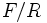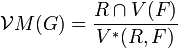# Baer invariant

## Definition

Suppose$G$ is a group. Suppose$\mathcal{V}$ is a subvariety of the variety of groups (note that$G$ may or may not be in$\mathcal{V}$). The Baer invariant of$G$ with respect to$\mathcal{V}$, denoted$\mathcal{V}M(G)$, is an abelian group defined as follows.

### Definition in terms of extensions

Consider possible group extensions of the form:$1 \to A \to E \to G \to 1$

satisfying the condition that the image of$A$ in$E$ is contained in the$\mathcal{V}$-marginal subgroup of$E$. Consider the set of defining words for$\mathcal{V}$ (note that it suffices to take any generating set of words for the variety). For each word$w$ with$n_w$ letters, we have a word map$E^{n_w} \to E$ (a set map only, not a homomorphism). There is a natural initial object dependent only on$G$ and$\mathcal{V}$ that has a unique homomorphism to the$\mathcal{V}$-verbal subgroup of$E$. This natural initial objection, which we will call$V^\#(G)$, has a unique homomorphism to the$\mathcal{V}$-verbal subgroup$V(G)$. The kernel of this homomorphism is the Baer invariant of$G$. PLACEHOLDER FOR INFORMATION TO BE FILLED IN: [SHOW MORE]

### Definition in terms of presentation

Suppose$G$ is expressed in the form$F/R$ where$F$ is a free group and$R$ is the normal closure of a set of words in$F$. Explicitly, any presentation of$G$ can be viewed in this manner, where$F$ is the free group on symbols corresponding to the generators and$R$ is the subgroup obtained as the normal closure of the relation words.

Denote by$V(F)$ the verbal subgroup of$F$ corresponding to all the words defining the variety$\mathcal{V}$, so$F/V(F)$ is the largest quotient of$F$ that is in$\mathcal{V}$. Also, define$V^*(R,F)$ as the subgroup generated by all words of the form:$v(f_1,f_2,\dots,f_ir,f_{i+1},\dots,f_n)v(f_1,f_2,\dots,f_n)^{-1}$

where$v$ varies over all words defining the variety$\mathcal{V}$,$f_1,f_2,\dots,f_n \in F$ (and$n,i$ are also free to vary) and$r$ varies over all of$R$.

Then, the Baer invariant of$G$ with respect to$\mathcal{V}$ is defined as:$\mathcal{V}M(G) = \frac{R \cap V(F)}{V^*(R,F)}$

## Particular cases

Case on subvariety Description of Baer invariant using presentation Other names and comments
abelian groups$\frac{R \cap [F,F]}{[R,F]}$ also called the Schur multiplier and denoted$M(G)$. See Hopf's formula for Schur multiplier.
nilpotent groups of class at most$c$$\frac{R \cap \gamma_{c+1}(F)}{[[ \dots[R,F],F],\dots],F]}$ ($F$ occurs$c$ times in the denominator) also called the$c$-nilpotent multiplier and denoted$M^{(c)}(G)$.